# 八、图像和视频处理

• 使用仿射和透视变换使图像变形
• 使用任意变换重新映射图像
• 背景减法
• 将许多图像拼接成全景图
• 使用非本地均值算法对照片降噪
• 构造 HDR 图像
• 通过图像修复消除照片中的缺陷

# 怎么做

1. 导入必要的模块，打开输入图像，然后复制它：
import cv2
import numpy as np

show_img = np.copy(img)

1. 定义两个函数来实现点选择过程：
selected_pts = []

def mouse_callback(event, x, y, flags, param):
global selected_pts, show_img

if event == cv2.EVENT_LBUTTONUP:
selected_pts.append([x, y])
cv2.circle(show_img, (x, y), 10, (0, 255, 0), 3)

def select_points(image, points_num):
global selected_pts
selected_pts = []

cv2.namedWindow('image')
cv2.setMouseCallback('image', mouse_callback)

while True:
cv2.imshow('image', image)

k = cv2.waitKey(1)

if k == 27 or len(selected_pts) == points_num:
break

cv2.destroyAllWindows()

return np.array(selected_pts, dtype=np.float32)

1. 选择图像中的三个点，使用cv2.getAffineTransform计算仿射变换，然后使用cv2.warpAffine应用仿射变换。 然后，显示结果图像：
show_img = np.copy(img)
src_pts = select_points(show_img, 3)
dst_pts = np.array([[0, 240], [0, 0], [240, 0]], dtype=np.float32)

affine_m = cv2.getAffineTransform(src_pts, dst_pts)

unwarped_img = cv2.warpAffine(img, affine_m, (240, 240))

cv2.imshow('result', np.hstack((show_img, unwarped_img)))
k = cv2.waitKey()

cv2.destroyAllWindows()

1. 找到一个仿射逆变换，将其应用并显示结果：
inv_affine = cv2.invertAffineTransform(affine_m)
warped_img = cv2.warpAffine(unwarped_img, inv_affine, (320, 240))

cv2.imshow('result', np.hstack((show_img, unwarped_img, warped_img)))
k = cv2.waitKey()

cv2.destroyAllWindows()

1. 使用cv2.getRotationMatrix2D创建一个旋转比例的仿射扭曲，并将其应用于图像：
rotation_mat = cv2.getRotationMatrix2D(tuple(src_pts), 6, 1)

rotated_img = cv2.warpAffine(img, rotation_mat, (240, 240))

cv2.imshow('result', np.hstack((show_img, rotated_img)))
k = cv2.waitKey()

cv2.destroyAllWindows()

1. 在图像中选择四个点，使用cv2.getPerspectiveTransform创建透视变形矩阵，然后将其应用于图像并显示结果：
show_img = np.copy(img)
src_pts = select_points(show_img, 4)
dst_pts = np.array([[0, 240], [0, 0], [240, 0], [240, 240]], dtype=np.float32)

perspective_m = cv2.getPerspectiveTransform(src_pts, dst_pts)

unwarped_img = cv2.warpPerspective(img, perspective_m, (240, 240))

cv2.imshow('result', np.hstack((show_img, unwarped_img)))
k = cv2.waitKey()

cv2.destroyAllWindows()


# 这个怎么运作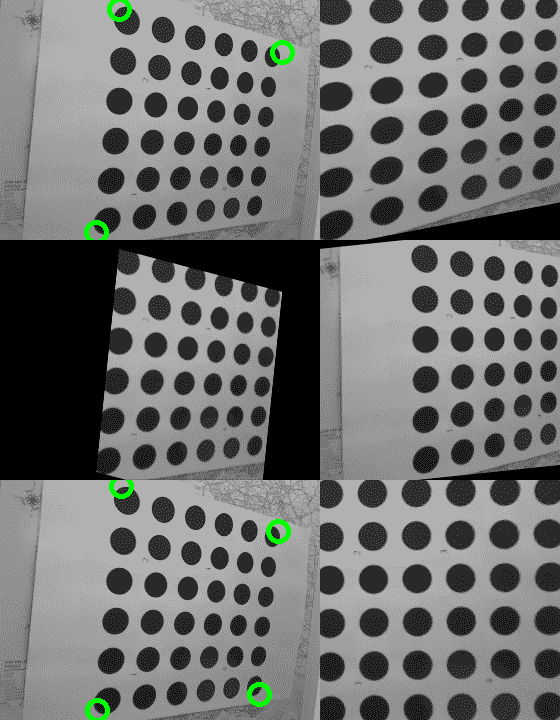# 怎么做

1. 导入必要的模块：
import math
import cv2
import numpy as np

1. 加载测试图像：
img = cv2.imread('../data/Lena.png')

1. 准备每个像素的变换图：
xmap = np.zeros((img.shape, img.shape), np.float32)
ymap = np.zeros((img.shape, img.shape), np.float32)
for y in range(img.shape):
for x in range(img.shape):
xmap[y,x] = x + 30 * math.cos(20 * x / img.shape)
ymap[y,x] = y + 30 * math.sin(20 * y / img.shape)

1. 重新映射源图像：
remapped_img = cv2.remap(img, xmap, ymap, cv2.INTER_LINEAR, None, cv2.BORDER_REPLICATE)

1. 可视化结果：
plt.figure(0)
plt.axis('off')
plt.imshow(remapped_img[:,:,[2,1,0]])
plt.show()


# 这个怎么运作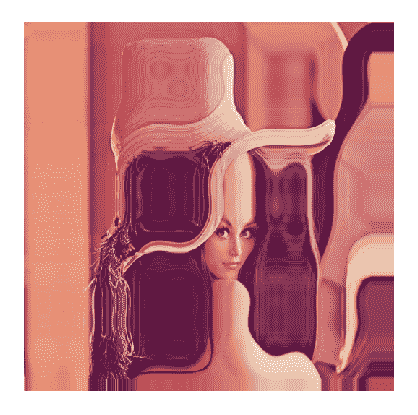# 怎么做

1. 导入必要的模块：
import cv2

1. 打开测试视频并初始化辅助变量：
video = cv2.VideoCapture('../data/traffic.mp4')
prev_pts = None
prev_gray_frame = None
tracks = None

1. 开始阅读视频中的帧，将每个图像转换为灰度：
while True:
if not retval: break
gray_frame = cv2.cvtColor(frame, cv2.COLOR_BGR2GRAY)

1. 使用稀疏的 Lucas-Kanade 光流算法跟踪上一帧的关键点，或者，如果您刚刚启动或按下C，请检测关键点，以便在下一帧中可以跟踪一些内容：
    if prev_pts is not None:
pts, status, errors = cv2.calcOpticalFlowPyrLK(
prev_gray_frame, gray_frame, prev_pts, None, winSize=(15,15), maxLevel=5,
criteria=(cv2.TERM_CRITERIA_EPS | cv2.TERM_CRITERIA_COUNT, 10, 0.03))
good_pts = pts[status == 1]
if tracks is None: tracks = good_pts
else: tracks = np.vstack((tracks, good_pts))
for p in tracks:
cv2.circle(frame, (p, p), 3, (0, 255, 0), -1)
else:
pts = cv2.goodFeaturesToTrack(gray_frame, 500, 0.05, 10)
pts = pts.reshape(-1, 1, 2)

1. 记住当前点和当前框架。 现在可视化结果并处理键盘输入：
    prev_pts = pts
prev_gray_frame = gray_frame

cv2.imshow('frame', frame)
key = cv2.waitKey() & 0xff
if key == 27: break
if key == ord('c'):
tracks = None
prev_pts = None

1. 关闭所有窗口：
cv2.destroyAllWindows()


# 这个怎么运作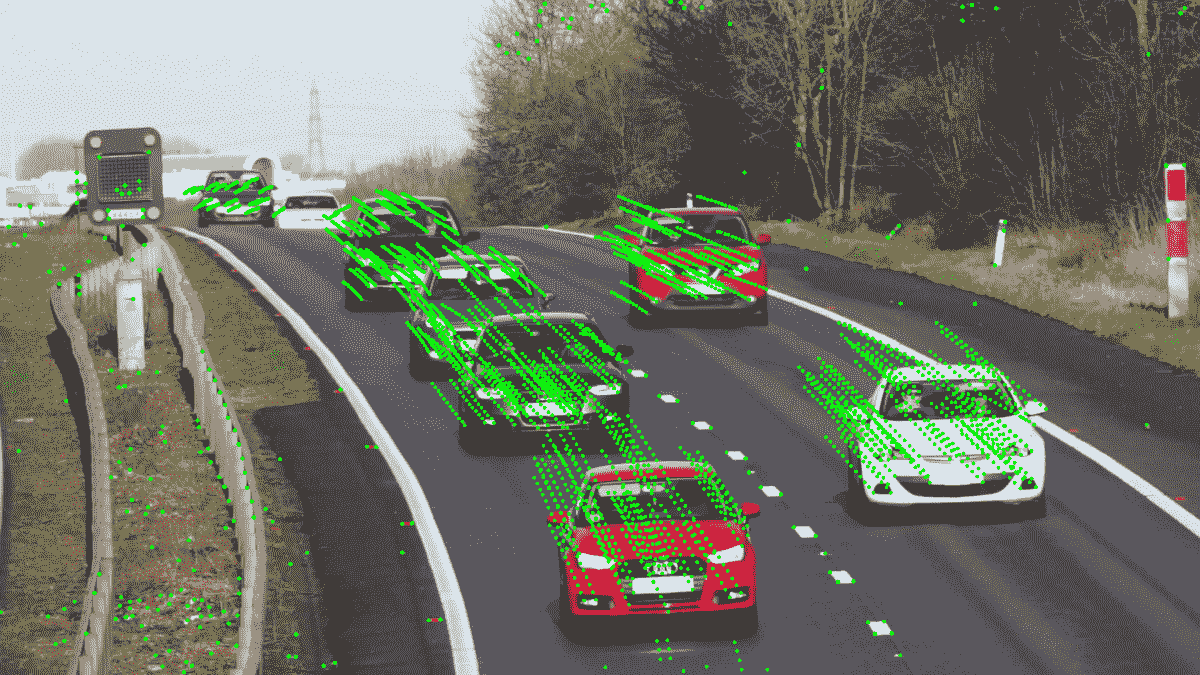# 怎么做

1. 导入必要的模块：
import cv2
import numpy as np

1. 定义一个打开视频文件的函数，并对每个帧应用一些背景减影算法：
def split_image_fgbg(subtractor, open_sz=(0,0), close_sz=(0,0), show_bg=False, show_shdw=False):
kernel_open = kernel_close = None

if all(i > 0 for i in open_sz):
kernel_open = cv2.getStructuringElement(cv2.MORPH_ELLIPSE, open_sz)

if all(i > 0 for i in close_sz):
kernel_close = cv2.getStructuringElement(cv2.MORPH_ELLIPSE, close_sz)

cap = cv2.VideoCapture('../data/traffic.mp4')
while True:
if not status_cap:
break

frame = cv2.resize(frame, None, fx=0.5, fy=0.5)

if kernel_open is not None:

if kernel_close is not None:
if kernel_open is not None:

foreground = frame

if show_shdw:

cv2.imshow('foreground', foreground)

if show_bg:
background = fgbg.getBackgroundImage()
if background is not None:
cv2.imshow('background', background)

if cv2.waitKey(30) == 27:
break

cap.release()
cv2.destroyAllWindows()

1. 将由 KadewTraKuPong 和 Bowden 创建的基于高斯混合的背景/前景分割算法应用于视频：
fgbg = cv2.bgsegm.createBackgroundSubtractorMOG()

split_image_fgbg(fgbg, (2, 2), (40, 40))

1. 创建由 Zoran Zivkovic 开发的高斯混合分割算法的改进版本的实例：
fgbg = cv2.createBackgroundSubtractorMOG2()

split_image_fgbg(fgbg, (3, 3), (30, 30), True)

1. 使用 Godbehere，Matsukawa 和 Goldberg 的背景减除算法创建背景遮罩：
fgbg = cv2.bgsegm.createBackgroundSubtractorGMG()

split_image_fgbg(fgbg, (5, 5), (25, 25))

1. 根据 Sagi Zeevi 的建议，应用基于计数的背景减法算法：
fgbg = cv2.bgsegm.createBackgroundSubtractorCNT()

split_image_fgbg(fgbg, (5, 5), (15, 15), True)

1. 使用基于最近邻方法的背景分割技术：
fgbg = cv2.createBackgroundSubtractorKNN()

split_image_fgbg(fgbg, (5, 5), (25, 25), True)


# 这个怎么运作

• cv2.BackgroundSubtractor.apply：获取分割遮罩
• cv2.BackgroundSubtractor.getBackgroundImage：检索背景图像

apply方法接受彩色图像作为参数并返回背景遮罩。 此遮罩通常包含三个值：0用于背景像素，255用于前景像素和127用于阴影像素。 阴影像素是背景中强度较低的像素。 值得一提的是，并非所有减法器都支持阴影像素分析。

getBackgroundImage返回背景图像，如果没有移动的物体，则应返回背景图像。 同样，只有少数减法器能够计算这样的图像。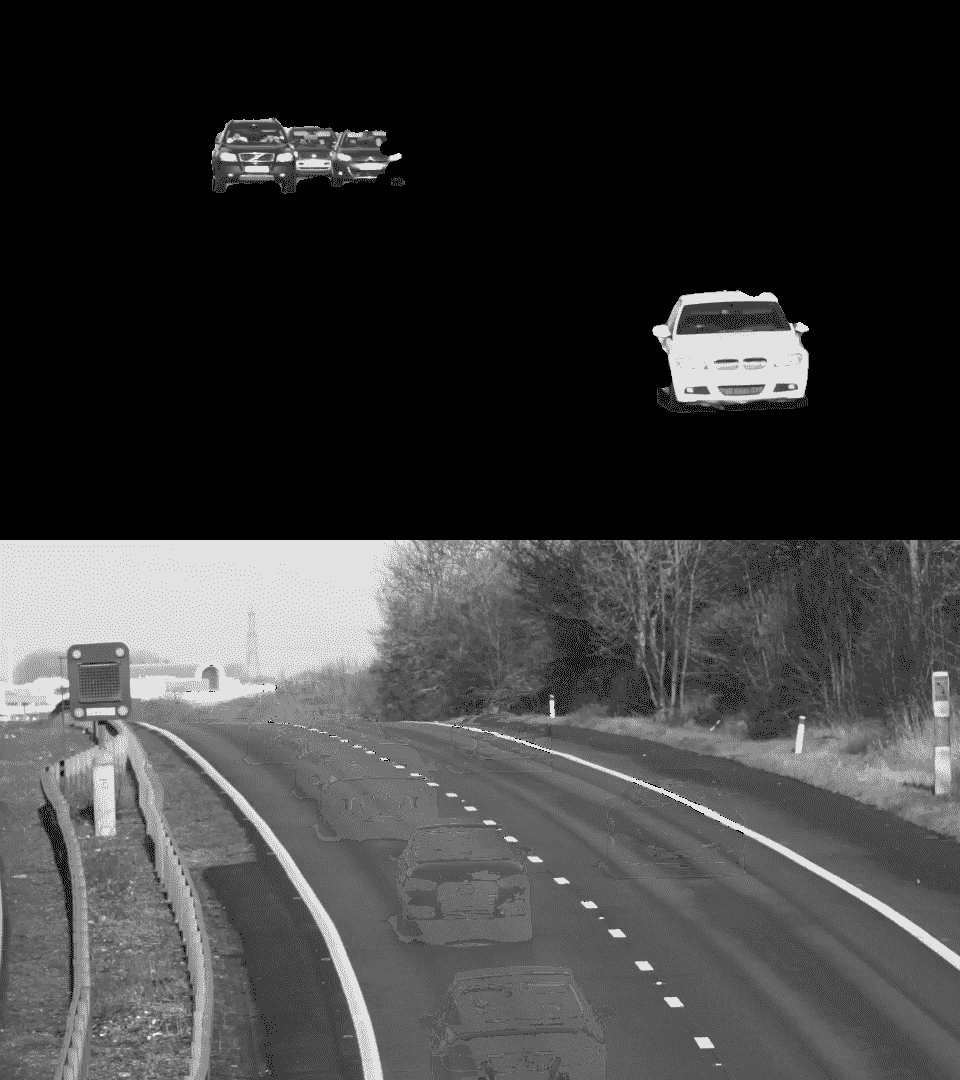# 将许多图像拼接成全景图

OpenCV 有很多计算机视觉算法。 其中一些是低级的，而另一些则在特殊情况下使用。 但是有功能，可以使用日常应用将许多算法结合在一起。 这些管道之一是全景拼接。 这个相当复杂的过程可以在 OpenCV 中轻松完成，并得到不错的结果。 此秘籍向您展示如何使用 OpenCV 工具创建自己的全景图。

# 怎么做

1. 导入必要的模块：
import cv2
import numpy as np

1. 加载我们将要合并为全景图的图像：
images = []

1. 创建一个全景拼接器，将图像传递给它，然后解析结果：
stitcher = cv2.createStitcher()
ret, pano = stitcher.stitch(images)

if ret == cv2.STITCHER_OK:
cv2.imshow('panorama', pano)
cv2.waitKey()

cv2.destroyAllWindows()
else:
print('Error during stiching')


# 这个怎么运作

cv2.createStitcher建立了全景拼接算法的实例。 要将其应用于全景图创建，您需要调用其stitch方法。 此方法接受要组合的图像数组，并返回拼接结果状态以及全景图像。 状态可能具有以下值之一：

• cv2.STITCHER_OK
• cv2.STITCHER_ERR_NEED_MORE_IMGS
• cv2.STITCHER_ERR_HOMOGRAPHY_EST_FAIL
• cv2.STITCHER_ERR_CAMERA_PARAMS_ADJUST_FAIL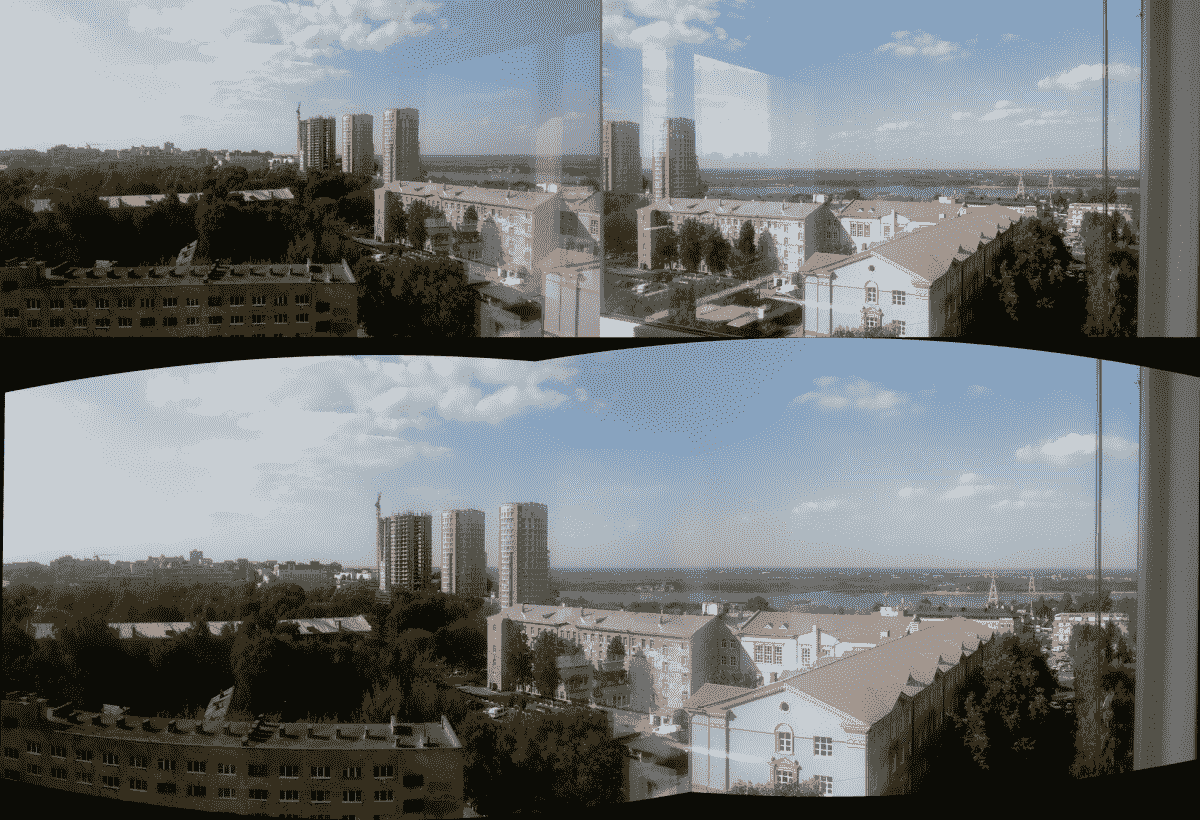# 怎么做

1. 导入必要的模块：
import cv2
import numpy as np
import matplotlib.pyplot as plt

1. 加载测试图像：
img = cv2.imread('../data/Lena.png')

1. 产生随机的高斯噪声：
noise = 30 * np.random.randn(*img.shape)
img = np.uint8(np.clip(img + noise, 0, 255))

1. 使用非本地均值算法执行去噪：
denoised_nlm = cv2.fastNlMeansDenoisingColored(img, None, 10)

1. 可视化结果：
plt.figure(0, figsize=(10,6))
plt.subplot(121)
plt.axis('off')
plt.title('original')
plt.imshow(img[:,:,[2,1,0]])
plt.subplot(122)
plt.axis('off')
plt.title('denoised')
plt.imshow(denoised_nlm[:,:,[2,1,0]])
plt.show()


# 这个怎么运作# 怎么做

1. 导入必要的模块：
import cv2
import numpy as np

1. 加载图像和曝光时间：
imgs_names = ['33', '100', '179', '892', '1560', '2933']

exp_times = []
images = []

for name in imgs_names:
exp_times.append(1/float(name))

exp_times = np.array(exp_times).astype(np.float32)

1. 恢复 CRF：
calibrate = cv2.createCalibrateDebevec()
response = calibrate.process(images, exp_times)

1. 计算 HDR 图像：
merge_debevec = cv2.createMergeDebevec()
hdr = merge_debevec.process(images, exp_times, response)

1. 将 HDR 图像转换为低动态范围LDR）图像以能够显示它：
tonemap = cv2.createTonemapDurand(2.4)
ldr = tonemap.process(hdr)

ldr = cv2.normalize(ldr, None, 0, 1, cv2.NORM_MINMAX)

cv2.imshow('ldr', ldr)
cv2.waitKey()
cv2.destroyAllWindows()

1. 应用此技术合并具有各种曝光度的图像：
merge_mertens = cv2.createMergeMertens()
fusion = merge_mertens.process(images)

fusion = cv2.normalize(fusion, None, 0, 1, cv2.NORM_MINMAX)

cv2.imshow('fusion', fusion)
cv2.waitKey()
cv2.destroyAllWindows()


# 这个怎么运作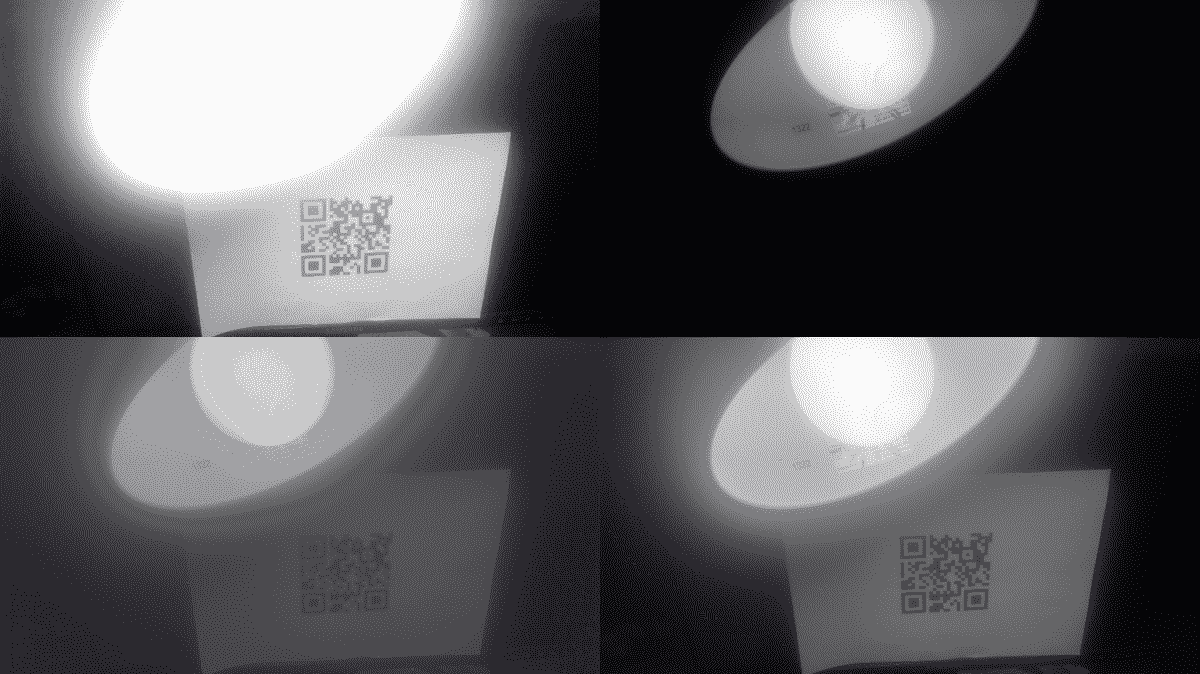# 怎么做

1. 导入必要的模块：
import cv2
import numpy as np

1. 定义一个封装掩码创建的类：
class MaskCreator:
self.prev_pt = None
self.image = image
self.dirty = False
self.show()

def show(self):

def mouse_callback(self, event, x, y, flags, param):
pt = (x, y)
if event == cv2.EVENT_LBUTTONDOWN:
self.prev_pt = pt
elif event == cv2.EVENT_LBUTTONUP:
self.prev_pt = None

if self.prev_pt and flags & cv2.EVENT_FLAG_LBUTTON:
cv2.line(self.image, self.prev_pt, pt, (127,)*3, 5)

self.dirty = True
self.prev_pt = pt
self.show()

1. 加载图像，创建其缺陷版本和遮罩，应用 Inpaint 算法，然后显示结果：
img = cv2.imread('../data/Lena.png')

defect_img = img.copy()

while True:
k = cv2.waitKey()
if k == 27:
break
if k == ord('a'):
res_telea = cv2.inpaint(defect_img, mask, 3, cv2.INPAINT_TELEA)
res_ns = cv2.inpaint(defect_img, mask, 3, cv2.INPAINT_NS)
cv2.imshow('TELEA vs NS', np.hstack((res_telea, res_ns)))
if k == ord('c'):
defect_img[:] = img
m_creator.show()
cv2.destroyAllWindows()


# 这个怎么运作

• 有缺陷的图像：它必须是 8 位彩色或灰度级图像
• 缺陷遮罩：它必须是 8 位单通道，并且必须与第一个参数中的图像大小相同
• 邻域半径损坏的像素周围区域的大小，应在计算其颜色时使用
• 修复模式：修复算法的类型